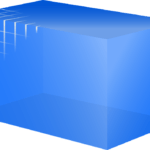Want to share your content on R-bloggers? click here if you have a blog, or here if you don't.There are 5 important basic data structures in R: vector, matrix, array, list and dataframe. They can be 1-dimensional (vector and list), 2-dimensional (matrix and data frame) or multidimensional (array). They also differ according to homogeneity of elements they can contain: while all elements contained in vector, matrix and array must be of the same type, list and data frame can contain multiple types.

In this set of exercises we shall practice casting between different types of these data structures, together with some basic operations on them. You can find more about data structures on Advanced R – Data structures page.

Answers to the exercises are available here.

If you have different solution, feel free to post it.

Exercise 1

Create a vector named v which contains 10 random integer values between -100 and +100.

Exercise 2

Create a two-dimensional 5×5 array named a comprised of sequence of even integers greater than 25.

Create a list named s containing sequence of 20 capital letters, starting with ‘C’.

Exercise 3

Create a list named l and put all previously created objects in it. Name them a, b and c respectively. How many elements are there in the list? Show the structure of the list. Count all elements recursively.

Exercise 4

Without running commands in R, answer the following questions:

1. what is the result of l[]?
2. How would you access random-th letter in the list element c?
3. If you convert list l to a vector, what will be the type of it’s elements?
4. Can this list be converted to an array? What will be the data type of elements in array?

Check the results with R.

Exercise 5

Remove letters from the list l. Convert the list l to a vector and check its class. Compare it with the result from exercise 4, question #3.

Exercise 6

Find the difference between elements in l[["a"]] and l[["b"]]. Find the intersection between them. Is there number 33 in their union?

Exercise 7

Create 5×5 matrix named m and fill it with random numeric values rounded to two decimal places, ranging from 1.00 to 100.00.

Exercise 8

Answer the following question without running R command, then check the result.

What will be the class of data structure if you convert matrix m to:

• vector
• list
• data frame
• array?

Exercise 9

Transpose array l\$b and then convert it to matrix.

Exercise 10

Get union of matrix m and all elements in list l and sort it ascending.